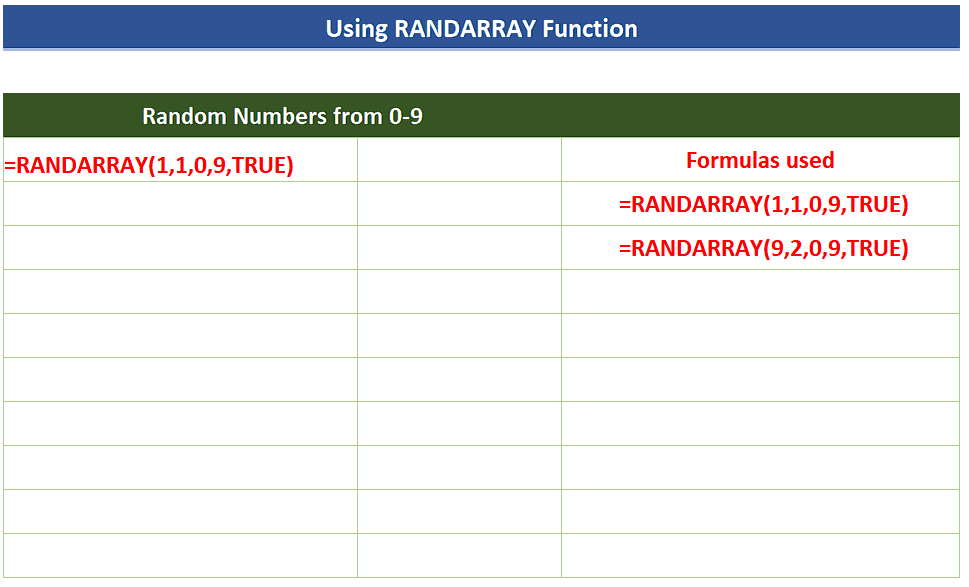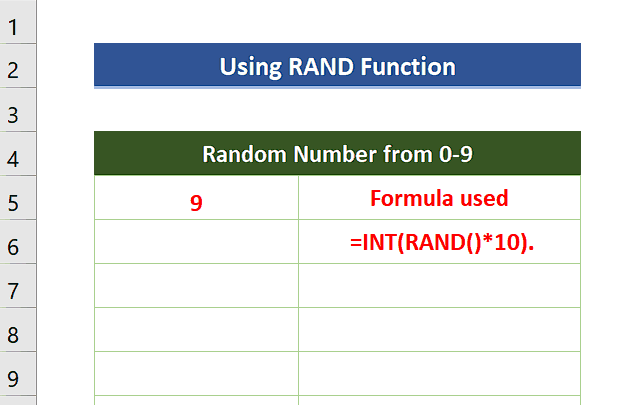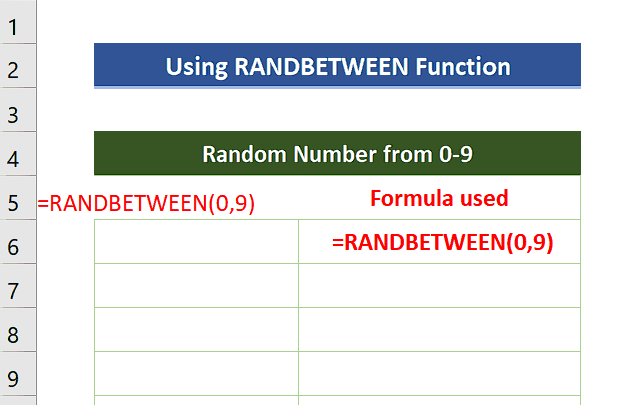# How to generate random number from 0 to 9 in Excel

In today’s tutorial we’ll learn how to generate random numbers from 0 to 9 in Excel using the following functions one by one.

• RAND
• RANDBETWEEN
• RANDARRAYExcel is a very powerful tool for data analysis and performing all sorts of calculations. Therefore, it provides us with a variety of tools to generate numbers in Excel. We can generate sequential as well as random numbers using built-in functions of Excel.

### Step 1 – Create the formula using RAND() to generate a random number from 0 to 9– As the RAND function generates a decimal random number greater than or equal to 0 and less than 1. So, we can’t simply use this function to generate a random number from 0 to 9. We’ll create a custom formula using RAND() function to achieve this goal. The custom formula is as follows;
=INT(RAND()*10))

– If we want to generate a list of random numbers from 0 to 9 then we can drag the formula down using the fill handle.

– Using the INT function before RAND and then multiplying it with 10 will generate an integer from 0 to 9. However, the number will change every time the sheet refreshes. Pressing F9 will refresh the sheet through a shortcut key and every time you press F9 the number will change its value.

### Step 2 – Use RANDBETWEEN() to generate a random number from 0 to 9– RANDBETWEEN function generates a random integer number between the numbers you specify.

– This time we can use the function without any modifications in it. The following formula will be used for the desired goal;
=RANDBETWEEN(0,9)

If we want to generate the list of random numbers you can always drag down the formula through the fill handle. Pressing F9 will refresh the sheet through a shortcut key and every time you press F9 the number will change its value as shown above.

### Step 3 – Use RANDARRAY() to generate random number from 0 to 9– The RANDARRAY function returns an array of random numbers. You can specify the number of rows and columns to fill, minimum and maximum values, and whether to return whole numbers or decimal values. The syntax is as follows;
=RANDARRAY([rows], [columns], [min], [max], [whole_number])

– The following formula will be used for the desired goal;
=RANDARRAY(1,1,0,9,TRUE)

The current formula will generate only 1 row and 1 column, i.e., only one entry by choosing 0 as minimum value and 9 as maximum value. Also choose TRUE for getting integer values.

If we want to generate the list of random numbers then change the values of rows and columns in the RANDARRAY function and use the formula mentioned below.
=RANDARRAY(9,2,0,9,TRUE)